International
Tables for
Crystallography
Volume C
Mathematical, physical and chemical tables
Edited by E. Prince

International Tables for Crystallography (2006). Vol. C, ch. 8.1, pp. 680-681

## Section 8.1.2. Principles of least squares

E. Princea and P. T. Boggsb

aNIST Center for Neutron Research, National Institute of Standards and Technology, Gaithersburg, MD 20899, USA, and bScientific Computing Division, National Institute of Standards and Technology, Gaithersburg, MD 20899, USA

### 8.1.2. Principles of least squares

| top | pdf |

The method of least squares may be formulated as follows: Given a set of n observations,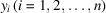, that are measurements of quantities that can be described by differentiable model functions,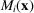, where x is a vector of parameters,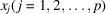, find the values of the parameters for which the sum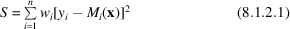is minimum. Here,represents a weight assigned to the ith observation. The values of the parameters that give the minimum value of S are called estimates of the parameters, and a function of the data that locates the minimum is an estimator. A necessary condition for S to be a minimum is for the gradient to vanish, which gives a set of simultaneous equations, the normal equations, of the form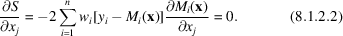The model functions,, are, in general, nonlinear, and there are no direct ways to solve these systems of equations. Iterative methods for solving them are discussed in Section 8.1.4. Much of the analysis of results, however, is based on the assumption that linear approximations to the model functions are good approximations in the vicinity of the minimum, and we shall therefore begin with a discussion of linear least squares.

To express linear relationships, it is convenient to use matrix notation. Let M(x) and y be column vectors whose ith elements are Mi(x) and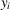. Similarly, let b be a vector and A be a matrix such that a linear approximation to the ith model function can be written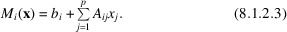Equations (8.1.2.3)can be written, in matrix form,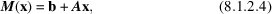and, for this linear model, (8.1.2.1)becomes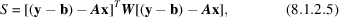where W is a diagonal matrix whose diagonal elements are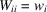. In this notation, the normal equations (8.1.2.2)can be written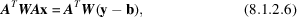and their solution is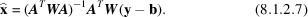If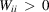for all i, and A has full column rank, then ATWA will be positive definite, and S will have a unique minimum at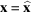. The matrix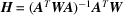is a p × n matrix that relates the n-dimensional observation space to the p-dimensional parameter space and is known as the least-squares estimator; because each element of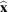is a linear function of the observations, it is a linear estimator. [Note that, in actual practice, the matrix H is not actually evaluated, except, possibly, in very small problems. Rather, the linear system ATWAx = ATW(yb) is solved using the methods of Section 8.1.3.]

The least-squares estimator has some special properties in statistical analysis. Suppose that the elements of y are experimental observations drawn at random from populations whose means are given by the model, M(x), for some unknown x, which we wish to estimate. This may be written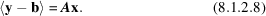The expected value of the least-squares estimate is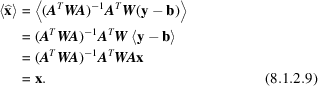If the expected value of an estimate is equal to the variable to be estimated, the estimator is said to be unbiased. Equation (8.1.2.9)shows that the least-squares estimator is an unbiased estimator for x, independent of W, provided only that y is an unbiased estimate of M(x), the matrix ATWA is nonsingular, and the elements of W are constants independent of y and M(x). Let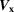and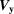be the variance–covariance matrices for the joint p.d.f.s of the elements of x and y, respectively. Then,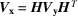. Let G be the matrix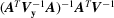, so that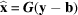is the particular least-squares estimate for which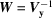. Then,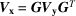. Ifis positive definite, its lower triangular Cholesky factor, L, exists, so that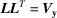. [If V is diagonal, L is also diagonal, with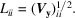] It is readily verified that the matrix product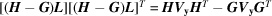, but the diagonal elements of this product are the sums of squares of the elements of rows of (HG)L, and are therefore greater than or equal to zero. Therefore, the diagonal elements of Vx, which are the variances of the marginal p.d.f.s of the elements of, are minimum when.

Thus, the least-squares estimator is unbiased for any positive-definite weight matrix, W, but the variances of the elements of the vector of estimated parameters are minimized if. [Note also that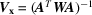if, and only if,.] For this reason, the least-squares estimator with weights proportional to the reciprocals of the variances of the observations is referred to as the best linear unbiased estimator for the parameters of a model describing those observations. (These specific results are included in a more general result known as the Gauss–Markov theorem.)

The analysis up to this point has assumed that the model is linear, that is that the expected values of the observations can be expressed by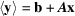, where A is some matrix. In crystallography, of course, the model is highly nonlinear, and this assumption is not valid. The principles of linear least squares can be extended to nonlinear model functions by first finding, by numerical methods, a point in parameter space,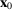, at which the gradient vanishes and then expanding the model functions about that point in Taylor's series, retaining only the linear terms. Equation (8.1.2.4)then becomes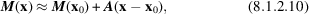where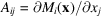evaluated at x = x0. Because we have already found the least-squares solution, the estimate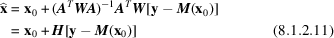reduces to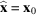. It is important, however, not to confuse, which is a convenient origin, with, which is a random variable describable by a joint p.d.f. with meanand a variance–covariance matrix, reducing to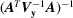when.

This variance–covariance matrix is the one appropriate to the linear approximation given in (8.1.2.10), and it is valid (and the estimate is unbiased) only to the extent that the approximation is a good one. A useful criterion for an adequate approximation (Fedorov, 1972) is, for each j and k,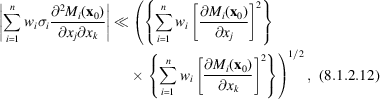where σi is the estimated standard deviation or standard uncertainty (Schwarzenbach, Abrahams, Flack, Prince & Wilson, 1995) of. This criterion states that the curvature of S(y, x) in a region whose size is of order σ in observation space is small; it ensures that the effect of second-derivative terms in the normal-equations matrix on the eigenvalues and eigenvectors of the matrix is negligible. [For a further discussion and some numerical tests of alternatives, see Donaldson & Schnabel (1986).]

The process of refinement can be viewed as the construction of a conditional p.d.f. of a set of model parameters, x, given a set of observations, y. An important expression for this p.d.f. is derived from two equivalent expressions for the joint p.d.f. of x and y: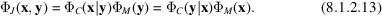Provided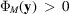, the conditional p.d.f. we seek can be written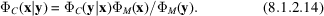Here, the factor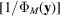is the factor that is required to normalize the p.d.f.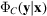is the conditional probability of observing a set of values of y as a function of x. When the observations have already been made, however, this can also be considered a density function for x that measures the likelihood that those particular values of y would have been observed for various values of x. It is therefore frequently written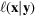, and (8.1.2.14)becomes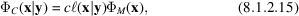where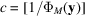is the normalizing constant.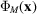, the marginal p.d.f. of x in the absence of any additional information, incorporates all previously available information concerning x, and is known as the prior p.d.f., or, frequently, simply as the prior of x. Similarly, ΦC(x|y) is the posterior p.d.f., or the posterior, of x. The relation in (8.1.2.14)and (8.1.2.15)was first stated in the eighteenth century by Thomas Bayes, and it is therefore known as Bayes's theorem (Box & Tiao, 1973). Although its validity has never been in serious question, its application has divided statisticians into two vehemently disputing camps, one of which, the frequentists, considers that Bayesian methods give nonobjective results, while the other, the Bayesians, considers that only by careful construction of a `noninformative' prior can true objectivity be achieved (Berger & Wolpert, 1984).

Diffraction data, in general, contain no phase information, so the likelihood function for the structure factor, F, given a value of observed intensity, will have a value significantly different from zero in an annular region of the complex plane with a mean radius equal to |F|. Because this is insufficient information with which to determine a crystal structure, a prior p.d.f. is constructed in one (or some combination) of two ways. Either the prior knowledge that electron density is non negative is used to construct a joint p.d.f. of amplitudes and phases, given amplitudes for all reflections and phases for a few of them (direct methods), or chemical knowledge and intuition are used to construct a trial structure from which structure factors can be calculated, and the phase of Fcalc is assigned to Fobs. Both of these procedures can be considered to be applications of Bayes's theorem. In fact, Fcalc for a refined structure can be considered a Bayesian estimate of F.

### References

Berger, J. O. & Wolpert, R. L. (1984). The likelihood principle. Hayward, CA: Institute of Mathematical Statistics.
Box, G. E. P. & Tiao, G. C. (1973). Bayesian inference in statistical analysis. Reading, MA: Addison-Wesley.
Donaldson, J. R. & Schnabel, R. B. (1986). Computational experience with confidence regions and confidence intervals for nonlinear least squares. Computer science and statistics. Proceedings of the Seventeenth Symposium on the Interface, edited by D. M. Allen, pp. 83–91. New York: North-Holland.
Fedorov, V. V. (1972). Theory of optimal experiments, translated by W. J. Studden & E. M. Klimko. New York: Academic Press.
Schwarzenbach, D., Abrahams, S. C., Flack, H. D., Prince, E. & Wilson, A. J. C. (1995). Statistical descriptors in crystallography. II. Report of a Working Group on Expression of Uncertainty in Measurement. Acta Cryst. A51, 565–569.